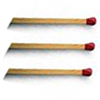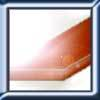#### You may also like### Construct-o-straws

Make a cube out of straws and have a go at this practical challenge.### Matchsticks

Reasoning about the number of matches needed to build squares that share their sides.### Little Boxes

How many different cuboids can you make when you use four CDs or DVDs? How about using five, then six?

# Right or Left?

##### Age 7 to 11Challenge Level

Many of you sent in correct answers to this problem, but not all were as well explained as these. Wilson from Beecroft Primary School wrote to say:

To work out the whether the dice are right- or left-handed, I pictured the dice in my head and then I matched them up with the pictures.

Philip from Woodfall Junior School also took this approach and says:

You can mentally rotate each die (finding the missing numbers by using the knowledge that opposite faces add up to seven) so that this happens, and then you can see if the die is right-handed, with the two at the front, or left-handed, with the three at the front.

April from Springfield Junior School explains exactly what she visualised:

1. Right-handed die
Roll it backwards so the $5$ is at the back and the $1$ is on the top. The front face will be $2$ because $5+2=7$. $3$ will still be on the right.

2. Right-handed die
Roll it to the right so the $6$ is on the bottom. That means $1$ is on the top because $6+1=7$. $3$ is on the right and $2$ stays at the front.

3. Right-handed die
Roll it backwards $2$ times so the $5$ is at the back and the 6 is on the bottom. The top is $1$ because $6+1=7$, and the front is $2$ because $5+2=7$. $3$ stays on the right.

4. Left-handed die
Roll it forwards so the $6$ is on the bottom. The top is $1$ because $6+1=7$. The $5$ moves from the top to the front. Turn it left so the five moves to the left hand side and the $3$ moves to the front. The right hand side is $2$ because $5+2=7$.

This is a great step-by-step way of approaching the problem, April. Well done.